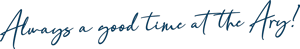Our GOOD TIMES RAFFLE is now

BIGGER and BETTER than ever!!!

More prizes!

Minimum prize value \$30!

\$200 Major Prize!

and more fun!!!!TICKETS

\$5 = 25 numbers

\$10 = 50 numbers

\$15 = 75 numbers

\$20 = 100 numbers + Bonus 50 numbers (\$10 worth)

\$25 = 125 numbers

\$30 = 150 numbers

\$35 = 175 numbers

\$40 = 200 numbers + Bonus 100 numbers (\$20 worth)

\$45 = 225 numbers

\$50 = 250 numbers

\$55 = 275 numbers

\$60 = 300 numbers + Bonus 150 numbers (\$30 worth)

\$65 = 325 numbers

\$70 = 350 numbers

\$75 = 375 numbers

\$80 = 400 numbers + bonus 200 numbers (\$40 worth)

\$85 = 425 numbers

\$90 = 450 numbers

\$95 = 475 numbers

\$100 = 500 numbers + bonus 250 numbers (\$50 worth)

**Please note Bonus Numbers only apply to cash sales

and aren't available in conjunction with any other offer.